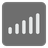Frequency Sorting
Elementary+
English RU

Your mission is to sort the list by the frequency of numbers included in it. If a few numbers have an equal frequency - they should be sorted according to their natural order. For example: [5, 2, 4, 1, 1, 1, 3] ==> [1, 1, 1, 2, 3, 4, 5]Input: Chaotic list of numbers.

Output: The list of numbers in which they are sorted by their frequency.

Example:

```frequency_sorting([5, 3, 8, 11, 5, 6, 6, 5]) == [5, 5, 5, 6, 6, 3, 8, 11]
```

How it is used: For analyzing data using mathematical statistics and mathematical analysis, and for finding trends and predicting future changes (systems, phenomena, etc.)

Precondition:
array length <= 100
max number <= 100